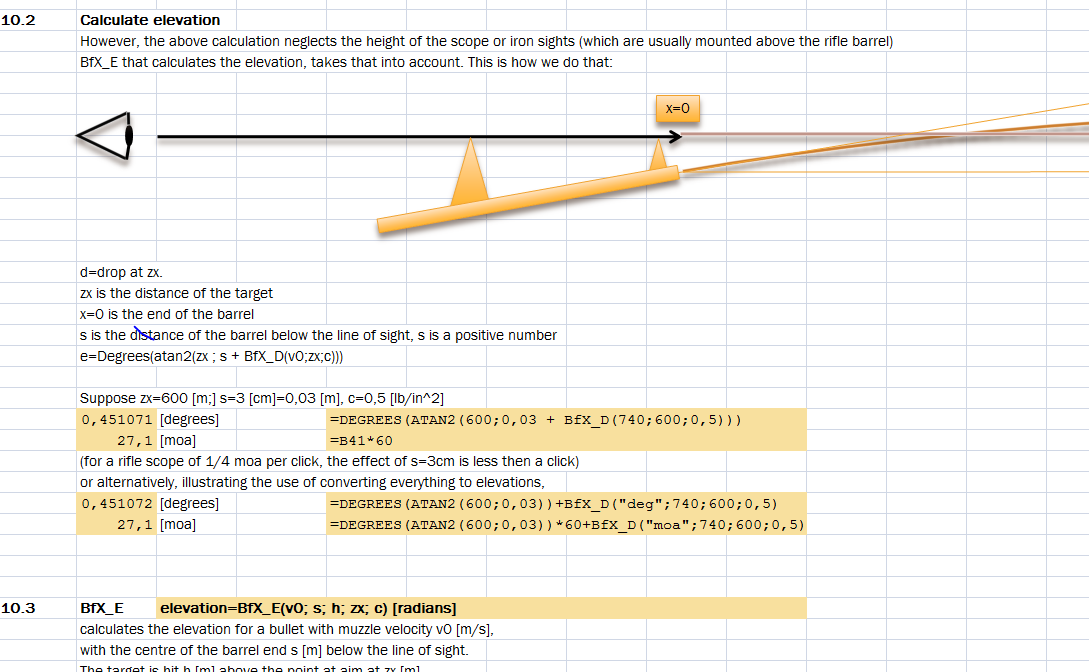# Forum

Home Ballistics for Excel Visit the BfX forum
HomeBfX supports metric and imperial in- and output units. It can also convert vertical (drop, path) and horizontal coordinates (wind deflection) of a trajectory to angles. Typically the minute of angle or MoA is used here, however (mili)radians for mildot calculations and  degrees are also possible. BfX functions are entered as a regular Excel function, e.g. =bfx_vx(740;300;0,5). Here the velocity of a bullet with a muzzle velocity of 740 m/s, at 300 m is calculated. The bullet has a G1 ballistic coefficient of 0,5 (or 0.5 depending on your country settings). The drag function used is the one of Arthur Pejsa, which is the default one which uses the G1 ballistic coefficient. ` =bfx_vx("fps";1200;"fps";300;"yd";0,25;"g7")` would calculate the velocity, in feet per second, for a muzzle velocity of  1200 fps at 300 yard for a bullet with a G7 ballistic coefficient of 0,25. Units can be mixed `=bfx_vx("fps";740;300;"yd";0,25;"g7")` would calculate the same for a 740 m/s muzzle velocity.